Select Page

# 12 Science CBSE Maths Answers for MCQ Relations and Functions in English

12 Science CBSE Maths Answers for MCQ Relations and Functions in English to enable students to get Answers in a narrative video format for the specific question.

Expert Teacher provides 12 Science CBSE Maths Answers for MCQ Relations and Functions through Video Answers in English language. This video solution will be useful for students to understand how to write an answer in exam in order to score more marks. This teacher uses a narrative style for a question from Relations and Functions not only to explain the proper method of answering question, but deriving right answer too.

Please find the question below and view the Answer in a narrative video format.

Question:

## Similar Questions from CBSE, 12th Science, Maths, Relations and Functions

Question 1 : Functions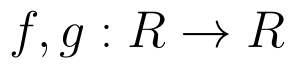are defined respectively, by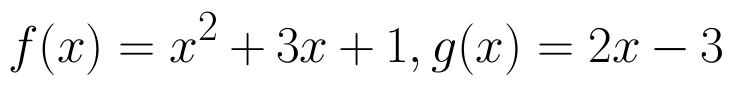, find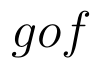(View Answer Video)

Question 2 : If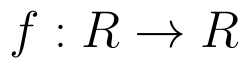is defined by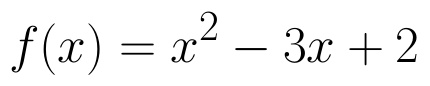, write f(f(x)). (View Answer Video)

Question 3 : Number of binary operations on the set { a, b} are: (View Answer Video)

Question 4 : If f(x) = |x| and g(x) = | 5x – 2 |. Then, fog = _____. (View Answer Video)

Question 5 : Let * be the binary operation on N given by a * b = LCM of a and b. Find 20 * 16. (View Answer Video)

### Application of Derivatives

Question 1 : The line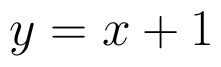is a tangent to the curve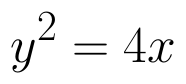at the point. (View Answer Video)

Question 2 : The normal  to the curve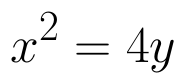passing (1,2) is____________. (View Answer Video)

Question 3 : What is the maximum value of the function sin x+ cos x? (View Answer Video)

Question 4 : Which of the following functions are strictly decreasing on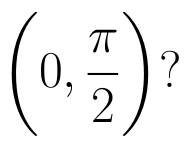(View Answer Video)

Question 5 : A tank with a rectangular base and rectangular sides, open at the top is to be constructed so that its depth is 2m and volume is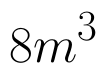If the building of tank costs Rs.70 per sq meter for the base and Rs.45 per sq meter for sides. What is the cost of least expensive? (View Answer Video)

### Vector Algebra

Question 1 : Find the direction of the vector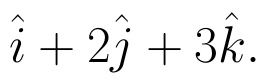(View Answer Video)

Question 2 :  Find the sum of the vectors :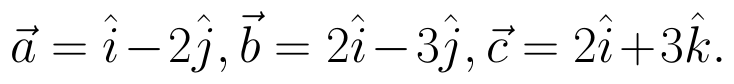(View Answer Video)

Question 3 : Find the value of x and y so that the vectors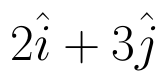and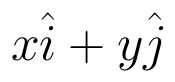are equal. (View Answer Video)

Question 4 :  Find the direction cosines of the vector joining the points A(1, 2, -3) and B(-1, -2, 1) directed from B to A. (View Answer Video)

Question 5 :  Write a unit vector in the direction of the sum of vectors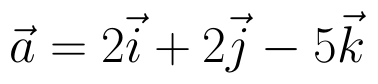and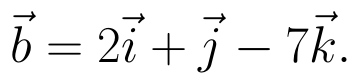(View Answer Video)

### Linear Programming

Question 1 : The objective function is maximum or minimum, which lies on the boundary of the feasible region. (View Answer Video)